Note: The other languages of the website are Google-translated. Back to English

## How to quickly calculate the overtime and payment in Excel?

For instance, there is a table recording a worker’s work on and work off time, and you want to calculate the overtime hour and the payment as below screenshot shown, how can you quickly solve this task in Excel?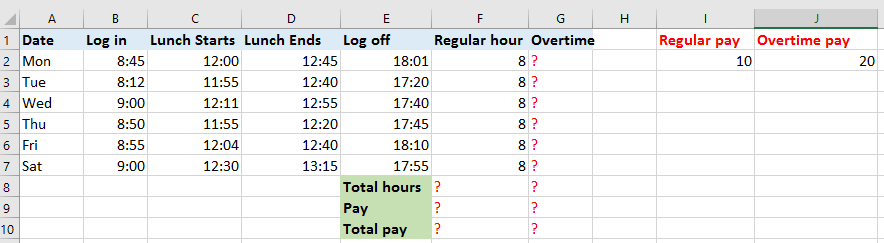Calculate overtime and payment

####Calculate overtime and payment

To calculate the overtime hour and payment, you can do as below:

1. Apply a formula to display the regular working hour first. In cell F1, enter this formula =IF((((C2-B2)+(E2-D2))*24)>8,8,((C2-B2)+(E2-D2))*24), and drag auto fill handle down to display the regular working hour of each day. See screenshot: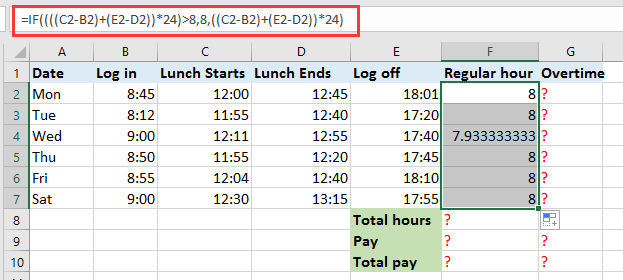2. In cell G2, which will display the overtime, enter this formula =IF(((C2-B2)+(E2-D2))*24>8, ((C2-B2)+(E2-D2))*24-8,0), drag AutoFill handle down to apply this formula to the cells, now the overtime of each day has been calculated. See screenshot: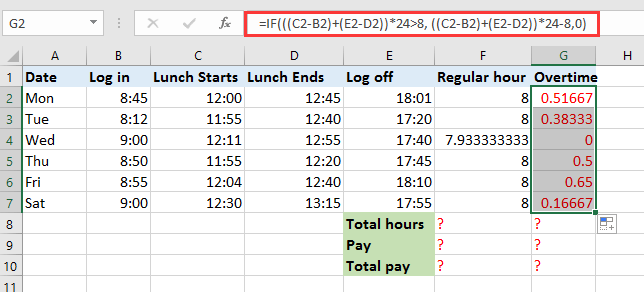In above formulas, C2 and D2 are the time that lunch starts and ends, and B2 and E2 are the time that log in and log off, 8 is the regular working hour each day, you can change these references as you need.

You can calculate the total working hours and payments.

3. In cell F8, type this formula =SUM(F2:F7) and drag to right to fill this formula to G8, now the total regular hours and overtime hours have been gotten.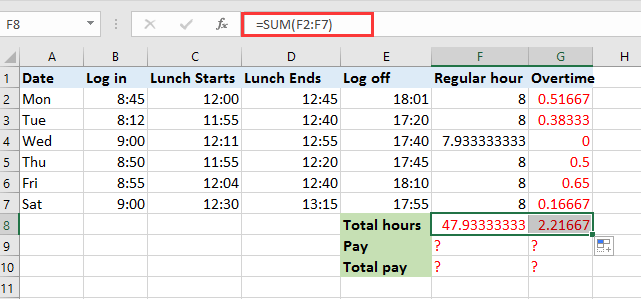4. In the cells which you want to calculate the payment for regular hours and overtime, enter the formula =F8*I2 and formula =G8*J2 separately, see screenshots:

In the formula, F8 and G8 are the cells containing total regular hours and total overtime, I2 and J2 contain the payment per hour.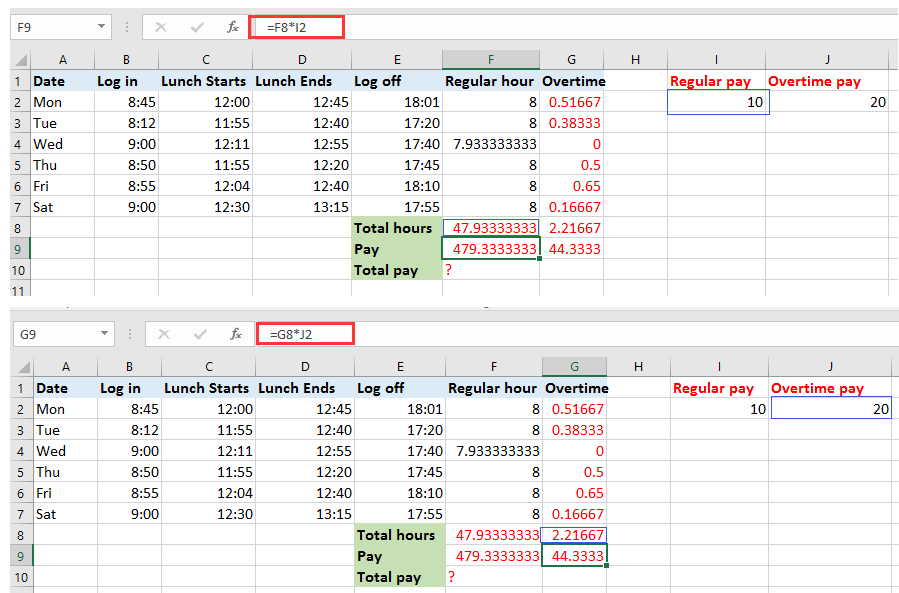You can apply =F9+G9 to calculate the total payments which include regular payments and overtime payment.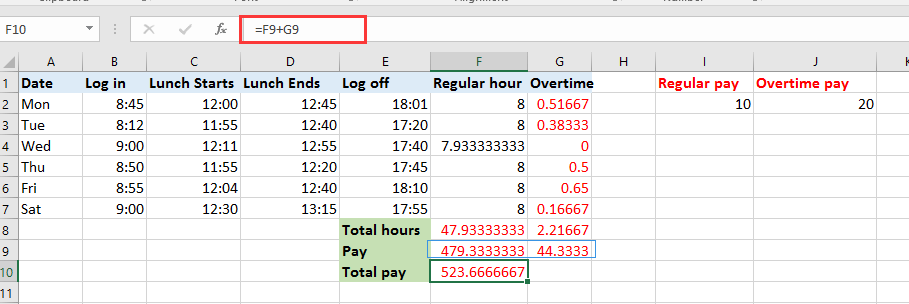#### Easily add days/years/month/hours/minutes/seconds to a datetime in Excel

Supposing you have a date time format data in a cell, and now you need to add a number of days, years, months, hours, minutes, or seconds to this date. Normally, using formula is the first method for all Excel users, but it’s hard to remember all formulas. With Kutools for Excel’s Date & Time Helper utility, you can easily add days, years, months, or hours, minutes or seconds to a date time, moreover, you can caculate the date difference, or the age based on a given birthday without remembering the formula at all. Click for full-featured free trial in 30 days!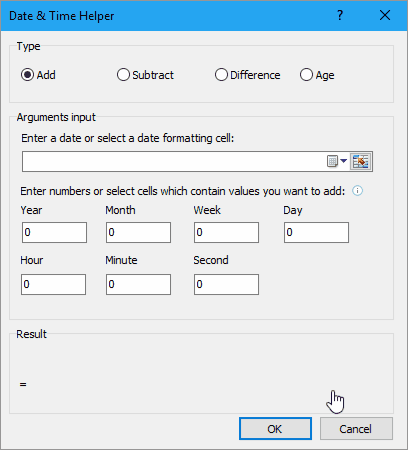### The Best Office Productivity Tools

#### Kutools for Excel Solves Most of Your Problems, and Increases Your Productivity by 80%

• Reuse: Quickly insert complex formulas, charts and anything that you have used before; Encrypt Cells with password; Create Mailing List and send emails...
• Super Formula Bar (easily edit multiple lines of text and formula); Reading Layout (easily read and edit large numbers of cells); Paste to Filtered Range...
• Merge Cells/Rows/Columns without losing Data; Split Cells Content; Combine Duplicate Rows/Columns... Prevent Duplicate Cells; Compare Ranges...
• Select Duplicate or Unique Rows; Select Blank Rows (all cells are empty); Super Find and Fuzzy Find in Many Workbooks; Random Select...
• Exact Copy Multiple Cells without changing formula reference; Auto Create References to Multiple Sheets; Insert Bullets, Check Boxes and more...
• Extract Text, Add Text, Remove by Position, Remove Space; Create and Print Paging Subtotals; Convert Between Cells Content and Comments...
• Super Filter (save and apply filter schemes to other sheets); Advanced Sort by month/week/day, frequency and more; Special Filter by bold, italic...
• Combine Workbooks and WorkSheets; Merge Tables based on key columns; Split Data into Multiple Sheets; Batch Convert xls, xlsx and PDF...
• More than 300 powerful features. Supports Office/Excel 2007-2019 and 365. Supports all languages. Easy deploying in your enterprise or organization. Full features 30-day free trial. 60-day money back guarantee.#### Office Tab Brings Tabbed interface to Office, and Make Your Work Much Easier

• Enable tabbed editing and reading in Word, Excel, PowerPoint, Publisher, Access, Visio and Project.
• Open and create multiple documents in new tabs of the same window, rather than in new windows.
• Increases your productivity by 50%, and reduces hundreds of mouse clicks for you every day!No ratings yet. Be the first to rate!
This comment was minimized by the moderator on the site
This was awesome should add the Double time calculations also. Thank you
This comment was minimized by the moderator on the site
hey so i have a timesheet for work i need it to calculate total hours, overtime 1 and overtime 2
where hours from 07:00-15:00 is normal hours so no overtime counted
hours from 15:00-17:00 is counted in overtime 1
and all other hours is counted in overtime 2
like so
if A1(start time)=07:00 B1(end time)=19:00 C1(total hours)=12 D1(overtime1)=2 E1(overtime2)=2
or A1(start time)=04:00 B1(end time)=16:00 C1(total hours)=12 D1(overtime1)=1 E1(overtime2)=3
This comment was minimized by the moderator on the site
How can I getting excel to calculate overtime in a template where the pay periods are first to the 15 and 16 through 31 st
There are no comments posted here yet